# We Helped With This Physics Homework: Have A Similar One?

SOLVEDCategory Physics Other College Solved Help On Physics Homework

## Short Assignment Requirements

I NEED ALL THE CORRECT ANSWERS TO THESEPROBLEMS. I DO NOT REQUIRE A DETAILEDEXPLANATION. BUT I NEED THE CORRECT ANSWERS.PLEASE WRITE ON THE PDF OF THE ASSIGNMENTAND DON'TPROVIDE SOLUTION ON A SEPARATE PIECE PAPER.

## Assignment Description

WebAssign

Homework #3 (Homework)

Current Score : 0.51 / 5          Due :1.                          0.17/0.17 points |  Previous AnswersOSGenChem1 4.1.P.002.

Consider molecular, net ionic, and complete ionic equations.

(a)    What is the difference between these types of equations?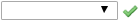Molecular   equations are written without regard to the dissociation of any ionic reactants or products, with all formulas represented as neutral substances. Complete ionic           equationsrepresent all dissolved ionic compounds with the formulas for their dissociated ions, including spectator ions. Net ionic    equations represent only those dissolved ions that are chemically or physically changed by the reaction, omitting any spectator ions.

(b)    In what circumstance would the complete and net ionic equations for a reaction be identical?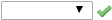If there are no        spectator ions involved in a reaction, its complete and net ionic equations will be the same.

2.                          0.17/0.17 points |  Previous AnswersOSGenChem1 4.1.P.004.

Balance the following equations. (Use the lowest possible whole number coefficients. Include states-ofmatter under the given conditions in your answer.)

(a)    Cr2O3(s) + SO3(l) Cr2(SO4)3(s)

 Cr2O3(s) + 3SO3(l) → Cr2(SO4)3(s) Cr_2O_3(s) + 3SO_3(l)-->Cr_2(SO_4)_3(s)

(b)    Bi(s) + H2O(l) Bi3O4(s) + H2(g)

 3Bi(s) + 4H2O(l)  →  Bi3O4(s) + 4H2(g) 3Bi(s) + 4H_2O(l) --> Bi_3O_4(s) + 4H_2(g)

(c)     N2(g) + O2(g) N2O4(g)

 N2(g) + 2O2(g)  →  N2O4(g) N_2(g) + 2O_2(g) --> N_2O_
 2In(s) + 3H2SO4(aq)  → In2(SO4)3(s) + 3H2(g) 2In(s) + 3H_2SO_4(aq) -->In_2(SO_4)_3(s) + 3H_2(g)

(d)    Sr3(PO4)2(aq) + H3PO4(aq) Sr(H2PO4)2(aq)

 Sr3(PO4)2(aq) +4H3PO4(aq)  → 3Sr(H2PO4)2(aq) Sr_3(PO_4)_2(aq) +4H_3PO_4(aq) -->3Sr(H_2PO_4)_2(aq)

(e)    MnCl4(s) + H2O(g) MnO2(s) + HCl(g)

 MnCl4(s) + 2H2O(g)  → MnO2(s) + 4HCl(g) MnCl_4(s) + 2H_2O(g) -->MnO_2(s) + 4HCl(g)

(f)     Au(s) + H2S(g) + O2(g) Au2S(s) + H2O(l)

 4Au(s) +2H2S(g) + O2(g)  →  2Au2S(s) +2H2O(l) 4Au(s) +2H_2S(g) + O_2(g) --> 2Au_2S(s) +2H_2O(l)

(g)    Pb(s) + H2O(l) + O2(g) Pb(OH)2(s)

 2Pb(s) + 2H2O(l) + O2(g)  →  2Pb(OH)2(s) 2Pb(s) + 2H_2O(l) + O_2(g) --> 2Pb(OH)_2(s)

(h)    In(s) + H2SO4(aq) In2(SO4)3(s) + H2(g)Supporting Materials

Periodic Table

Constants and Factors Supplemental DataSection 4.1

3.                          OSGenChem1 4.1.P.006.

Write a balanced equation describing each of the following chemical reactions. (Use the lowest possible whole number coefficients. Include states-of-matter under the given conditions in your answer.)

(a)    Solid potassium bromate, KBrO3, decomposes to form solid potassium bromide and diatomic oxygen gas.

(b)    Solid gallium metal reacts with solid diatomic iodine to form solid Ga2I6.

(c)     When solid potassium chloride is added to aqueous sulfuric acid, hydrogen chloride gas and aqueous potassium sulfate are produced.

(d)    Aqueous solutions of phosphoric acid and rubidium hydroxide react to produce aqueous rubidium dihydrogen phosphate and liquid water.

Supporting Materials

Periodic Table

Constants and Factors Supplemental DataSection 4.1

4.                          0.17/0.17 points |  Previous AnswersOSGenChem1 4.1.P.008.

Write the chemical formula for a covalent compound that will balance the equation. (Omit states-of-matter from your answer.)

C3H6O2 + NaOH C3H5O2 + Na+ + ?

 H2O H_2O

5.                          OSGenChem1 4.1.P.010.

A novel process for obtaining magnesium from sea water involves several reactions. Write a balanced chemical equation for each step of the process. (Use the lowest possible whole number coefficients. Include states-of-matter under the given conditions in your answer.)

(a)    The first step is the decomposition of solid calcium carbonate from seashells to form solid calcium oxide and gaseous carbon dioxide.

(b)    The second step is the formation of solid calcium hydroxide as the only product from the reaction of the solid calcium oxide with liquid water.

(c)     In the third step, calcium hydroxide (mixed with seawater) reacts with dissolved magnesium chloride to yield solid magnesium hydroxide and aqueous calcium chloride.

(d)    In the fourth step, solid magnesium hydroxide is added to an aqueous hydrochloric acid solution, producing dissolved magnesium chloride and liquid water.

(e)    Finally, the magnesium chloride is melted and this liquid is electrolyzed to yield liquid magnesium metal and diatomic chlorine gas.

6.                          OSGenChem1 4.1.WA.001.

One mole of propane (C3H8) gas reacts with five moles of oxygen (O2) gas to produce three moles of carbon dioxide gas (CO2) and four moles of water gas (H2O). Using this description of the reaction, write the corresponding chemical equation, showing the appropriate coefficients for each reactant and product. (Include states-of-matter under the given conditions in your answer.)

Supporting Materials

Periodic Table

Constants and Factors Supplemental DataSection 4.1 Tutorial7. –/0.17 pointsOSGenChem1 4.1.WA.003.

Determine the number of molecules of oxygen (O2) required to complete the following chemical equation.

N2 + O2 2 NO2

The number of molecules of oxygen (O2) required is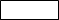.

Supporting Materials

Periodic Table

Constants and Factors Supplemental DataSection 4.1

Tutorial

8.                    OSGenChem1 4.1.WA.004.

Methane gas (CH4) reacts with fluorine gas (F2) to produce gaseous carbon tetrafluoride (CF4) and hydrogen fluoride gas (HF). The balanced chemical equation for the reaction is as follows:

CH4(g) + 4 F2(g) CF4(g) + 4 HF(g).

Calculate the number and types of atoms present in the reactants in this chemical equation.C

F

H

Supporting Materials

Periodic Table

Constants and Factors Supplemental DataSection 4.1 Tutorial9. –/0.17 pointsOSGenChem1 4.1.WA.007.

The combustion of butane gas (C4H10) involves the reaction of butane with oxygen (O2) to produce carbon dioxide (CO2) and water (H2O). The chemical equation is as follows:

2 C4H10(g) + 13 O2(g) 8 CO2(g) + 10 H2O(g). Identify the reactants and products from the following.

(a)  the descriptive statement of the reaction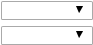The ---Select---       are butane and oxygen.

The ---Select---      are carbon dioxide and water.

(b)  the chemical equation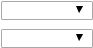The ---Select---       are C4H10 and O2.

The ---Select---       are CO2 and H2O.

Supporting Materials

Periodic Table

Constants and Factors Supplemental DataSection 4.1

Tutorial

10.–                 OSGenChem1 4.1.WA.011.

Aluminum reacts with hydrochloric acid to produce aluminum chloride and hydrogen gas. The reaction may be represented by the following unbalanced chemical equation:

2 Al(s) + 6 HCl(aq) 2 AlCl3(aq) + x H2(g).

What minimum value of the coefficient x would balance the equation?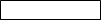Supporting Materials

Periodic Table

Constants and Factors Supplemental DataSection 4.1 Tutorial11.–/0.17 pointsOSGenChem1 4.1.WA.013.

Balance the following reaction, using the smallest integer coefficients that correctly represent the stoichiometric ratios in the balanced reaction. (Omit states-of-matter from your answer.)

C4H8 + O2 CO2 + H2O

Supporting Materials

Periodic Table

Constants and Factors Supplemental DataSection 4.1

Tutorial

12.–                 OSGenChem1 4.1.WA.014.

Beryllium carbide (Be2C) reacts with water (H2O) to produce methane (CH4) and beryllium hydroxide (Be(OH)2). The unbalanced chemical equation for this reaction can be written as the following.

Be2C + H2O CH4 + Be(OH)2 Balance the chemical equation.

Supporting Materials

Periodic Table

Constants and Factors Supplemental DataSection 4.1 Tutorial13.–/0.17 pointsOSGenChem1 4.1.WA.017.

When aqueous solutions of lead(II) nitrate (Pb(NO3)2) and potassium phosphate (K3PO4) are mixed, the products are solid lead(II) phosphate and aqueous potassium nitrate. Write the balanced chemical equation for this reaction. (Include states-of-matter under the given conditions in your answer. Use the lowest possible whole number coefficients.)

Supporting Materials

Periodic Table

Constants and Factors Supplemental DataSection 4.1

Tutorial

14.–                 OSGenChem1 4.1.WA.022.

Write the net ionic equation for the reaction of aqueous solutions of silver perchlorate and sodium iodide. (Include states-of-matter under the given conditions in your answer. Use the lowest possible whole number coefficients.)

Supporting Materials

Periodic Table

Constants and Factors Supplemental DataSection 4.1 Tutorial15.–/0.17 pointsOSGenChem1 4.1.WA.023.

Identify the spectator ion(s) in the following complete (total) ionic equation. (Separate substances in a list with a comma. Omit states-of-matter from your answer.)

Pb2+(aq) + 2 NO3(aq) + 2 K+(aq) + 2 I(aq) PbI2(s) + 2 K+(aq) + 2 NO3(aq)

Supporting Materials

Periodic Table

Constants and Factors Supplemental DataSection 4.1

Tutorial

16.–                 OSGenChem1 4.2.P.012.

Use the following equations to answer the next four questions.

i.    H2O(l) H2O(g)

ii.   Rb+(aq) + Cl(aq) + Cu+(aq) + NO3(aq) CuCl(s) + Rb+(aq) + NO3(aq)

iii.  CH3CH2CH2OH(g) + O2(g) CO2(g) + H2O(g)

iv.  2 H2O(l) 2 H2(g) + O2(g)

v.   Ti2+(aq) + 2 OH(aq) Ti(OH)2(s)

(a)     Which equation describes a physical change?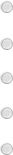H2O(l) H2O(g)

Rb+(aq) + Cl(aq) + Cu+(aq) + NO3(aq) CuCl(s) + Rb+(aq) + NO3(aq)

CH3CH2CH2OH(g) + O2(g) CO2(g) + H2O(g)

2 H2O(l) 2 H2(g) + O2(g)

Ti2+(aq) + 2 OH(aq) Ti(OH)2(s)

(b)     Which equation identifies the reactants and products of a combustion reaction?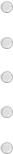H2O(l) H2O(g)

Rb+(aq) + Cl(aq) + Cu+(aq) + NO3(aq) CuCl(s) + Rb+(aq) + NO3(aq)

CH3CH2CH2OH(g) + O2(g) CO2(g) + H2O(g)

2 H2O(l) 2 H2(g) + O2(g) Ti2+(aq) + 2 OH(aq) Ti(OH)2(s)

(c)     Which equation is not balanced?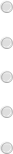H2O(l) H2O(g)

Rb+(aq) + Cl(aq) + Cu+(aq) + NO3(aq) CuCl(s) + Rb+(aq) + NO3(aq)

CH3CH2CH2OH(g) + O2(g) CO2(g) + H2O(g)

2 H2O(l) 2 H2(g) + O2(g) Ti2+(aq) + 2 OH(aq) Ti(OH)2(s)

(d)     Which is a net ionic equation?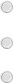H2O(l) H2O(g)

Rb+(aq) + Cl(aq) + Cu+(aq) + NO3(aq) CuCl(s) + Rb+(aq) + NO3(aq)

CH3CH2CH2OH(g) + O2(g) CO2(g) + H2O(g)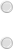2 H2O(l) 2 H2(g) + O2(g)

Ti2+(aq) + 2 OH(aq) Ti(OH)2(s)

17.–                 OSGenChem1 4.2.P.016.

Determine the oxidation states of the elements in the following compounds. (Enter your answer using the format +1 and -2.)

(a) H2S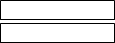H S

 (b) CaC2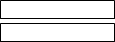Ca

C

(c)     NaBH4Na

B

H

(d)    BaO2 (barium peroxide)Ba O

 (e) NaHNa H

 (f) FeF3Fe

F

 (g) HClH

Cl

Supporting Materials

Periodic Table

Constants and Factors Supplemental DataSection 4.218.–/0.17 pointsOSGenChem1 4.2.P.020a.

Identify the atoms that are oxidized and reduced, the change in oxidation state for each, and the oxidizing and reducing agents in the following equation.

Mg(s) + NiCl2(aq) MgCl2(aq) + Ni(s)

 oxidized or reduced? oxidation state as reactant oxidation state as product Mg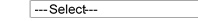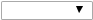---Select---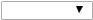---Select--- Ni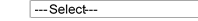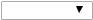---Select---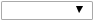---Select--- Cl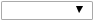---Select---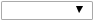---Select---

Oxidizing agent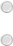Mg

NiCl2

Reducing agent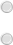Mg

NiCl2

19.–                OSGenChem1 4.2.P.020b.

Identify the atoms that are oxidized and reduced, the change in oxidation state for each, and the oxidizing and reducing agents in the following equation.

PCl3(l) + Cl2(g) PCl5(s)

 oxidized or reduced? oxidation state as reactant oxidation state as product P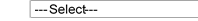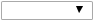---Select---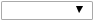---Select--- Cl in/from PCl3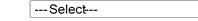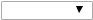---Select---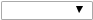---Select--- Cl in/from Cl2---Select------Select---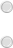Oxidizing agent PCl3

Cl2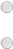Reducing agent PCl3

Cl2

20.–                 OSGenChem1 4.2.P.022.

Complete and balance the following acid-base equations. (Assume that these reactions go to completion. Use the lowest possible whole number coefficients. Include states-of-matter under the given conditions in your answer.)

(a)    Solid Fe2O3 is treated with a solution of H2SO4

(b)    Solid Zn(OH)2 is treated with a solution of HNO3.

(c)     A solution of H3AsO4 reacts with solid NaOH.21.–/0.17 pointsOSGenChem1 4.2.P.030.

In a common experiment in the general chemistry laboratory, magnesium metal is heated in air to produce MgO. MgO is a white solid, but in these experiments it often looks gray, due to small amounts of Mg3N2, a compound formed as some of the magnesium reacts with nitrogen. Write a balanced equation for each reaction. (Include states-of-matter under the given conditions in your answer. Use the lowest possible whole number coefficients.) Magnesium reacts with oxygen

Magnesium reacts with nitrogen

22.–                 OSGenChem1 4.2.P.032.

Calcium propionate is sometimes added to bread to retard spoilage. This compound can be prepared by the reaction of calcium carbonate, CaCO3, with propionic acid, HCH5CO2, which has properties similar to those of acetic acid. Write the balanced equation for the formation of calcium propionate. (Include states-ofmatter under the given conditions in your answer. Use the lowest possible whole number coefficients.)

23.–/0.17 pointsOSGenChem1 4.2.P.034.

Solid copper(II) sulfide is oxidized by molecular oxygen to produce gaseous sulfur trioxide and solid copper(II) oxide. The gaseous product then reacts with liquid water to produce liquid sulfuric acid as the only product. Write the two equations which represent these reactions. (Include states-of-matter under the given conditions in your answer. Use the lowest possible whole number coefficients.) 1st reaction step

24.–                 OSGenChem1 4.2.P.038.

Complete and balance each of the following half-reactions. (Include states-of-matter under the given conditions in your answer. Use the lowest possible whole number coefficients. For the purpose of this exercise, protons need to be written as H+ instead of H3O+, unless prompted otherwise.)

(a)     Cr2+(aq) Cr3+(aq)

(b)     Hg(l) + Br(aq) HgBr42(aq)

(c)     ZnS(s) Zn(s) + S2(aq)

(d)     H2(g) H2O(l) (in basic solution)

(e)     H2(g) H3O+(aq) (in acidic solution)

(f)      NO3(aq) HNO2(aq) (in acidic solution)

(g)     MnO2(s) MnO4(aq) (in basic solution)

(h)    Cl(aq) ClO3(aq) (in acidic solution)

25.–                 OSGenChem1 4.2.P.040.

Balance each of the following equations according to the half-reaction method. (Include states-of-matter under the given conditions in your answer. Use the lowest possible whole number coefficients. For the purpose of this exercise, protons need to be written as H+ instead of H3O+.)

(a)     Zn(s) + NO3(aq) Zn2+(aq) + N2(g) (in acidic solution)

(b)     Zn(s) + NO3(aq) Zn2+(aq) + NH3(aq) (in basic solution)

(c)     CuS(s) + NO3(aq) Cu2+(aq) + S(s) + NO(g) (in acidic solution)

(d)     NH3(aq) + O2(g) NO2(g) (in gas phase)

(e)     Cl2(g) + OH(aq) Cl(aq) + ClO3(aq) (in basic solution)

(f)      H2O2(aq) + MnO4(aq) Mn2+(aq) + O2(g) (in acidic solution)

(g)     NO2(g) NO3(aq) + NO2(aq) (in basic solution)

(h)    Fe3+(aq) + I(aq) Fe2+(aq) + I2(aq) (in solution)

Supporting Materials

Periodic Table

Constants and Factors Supplemental DataSection 4.2

26.–                 OSGenChem1 4.2.WA.005.

Summarize the solubility guidelines for common ions. (This question is graded according to a set of solubility rules provided by WebAssign. The solubility rules provided with your textbook might be slightly different. Select all that apply.)

All nitrates and acetates are soluble, except for the following ions.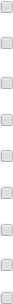Ag+

Hg22+

Pb2+

Ca2+

Sr2+

Ba2+

NH4+

alkali metals

There are no known exceptions.

All chlorides, bromides, and iodides are soluble, except for the following ions.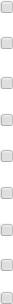Ag+

Hg22+

Pb2+

Ca2+

Sr2+

Ba2+

NH4+

alkali metals

There are no known exceptions.

All sulfates are soluble, except for the following ions.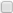Ag+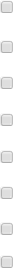Hg22+

Pb2+

Ca2+

Sr2+

Ba2+

NH4+

alkali metals

There are no known exceptions.

All sulfides are insoluble, except for the following ions.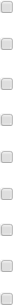Ag+

Hg22+

Pb2+

Ca2+

Sr2+

Ba2+

NH4+

alkali metals

There are no known exceptions.

All carbonates are insoluble, except for the following ions.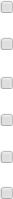Ag+

Hg22+

Pb2+

Ca2+

Sr2+

Ba2+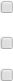NH4+

alkali metals

There are no known exceptions.

All phosphates are insoluble, except for the following ions.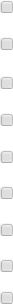Ag+

Hg22+

Pb2+

Ca2+

Sr2+

Ba2+

NH4+

alkali metals

There are no known exceptions.

All hydroxides are insoluble, except for the following ions.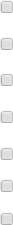Ag+

Pb2+

Ca2+

Sr2+

Ba2+

alkali metals

There are no known exceptions.

Supporting Materials

Periodic Table

Constants and Factors

Supplemental DataSection 4.2 Tutorial27.–/0.17 pointsOSGenChem1 4.2.WA.011.

Determine the oxidation number of V in the V5O143− ion given that the oxidation number of oxygen isSupporting Materials

Periodic Table

Constants and Factors Supplemental DataSection 4.2

Tutorial

28.–/0.41 pointsOSGenChem1 4.2.WA.014.

Write the oxidation numbers of the following. (Enter your answer using the format +1 and -2.)

(a)       F in F2(b)       N in NH4+(c)       V in V2O74−(d)       Co in Co(NO3)2Supporting Materials

Periodic Table

Constants and Factors Supplemental DataSection 4.2

Tutorial

Strategy Video

 nd reaction step

Is it free to get my assignment evaluated?

Yes. No hidden fees. You pay for the solution only, and all the explanations about how to run it are included in the price. It takes up to 24 hours to get a quote from an expert. In some cases, we can help you faster if an expert is available, but you should always order in advance to avoid the risks. You can place a new order here.

How much does it cost?

The cost depends on many factors: how far away the deadline is, how hard/big the task is, if it is code only or a report, etc. We try to give rough estimates here, but it is just for orientation (in USD):

 Regular homework \$20 - \$150 Advanced homework \$100 - \$300 Group project or a report \$200 - \$500 Mid-term or final project \$200 - \$800 Live exam help \$100 - \$300 Full thesis \$1000 - \$3000

How do I pay?

Credit card or PayPal. You don't need to create/have a Payal account in order to pay by a credit card. Paypal offers you "buyer's protection" in case of any issues.

Why do I need to pay in advance?

We have no way to request money after we send you the solution. PayPal works as a middleman, which protects you in case of any disputes, so you should feel safe paying using PayPal.

Do you do essays?

No, unless it is a data analysis essay or report. This is because essays are very personal and it is easy to see when they are written by another person. This is not the case with math and programming.

Why there are no discounts?

It is because we don't want to lie - in such services no discount can be set in advance because we set the price knowing that there is a discount. For example, if we wanted to ask for \$100, we could tell that the price is \$200 and because you are special, we can do a 50% discount. It is the way all scam websites operate. We set honest prices instead, so there is no need for fake discounts.

Do you do live tutoring?

No, it is simply not how we operate. How often do you meet a great programmer who is also a great speaker? Rarely. It is why we encourage our experts to write down explanations instead of having a live call. It is often enough to get you started - analyzing and running the solutions is a big part of learning.

What happens if I am not satisfied with the solution?

Another expert will review the task, and if your claim is reasonable - we refund the payment and often block the freelancer from our platform. Because we are so harsh with our experts - the ones working with us are very trustworthy to deliver high-quality assignment solutions on time.

## Popular Solved Assignments Like This

Customer Feedback

"Thanks for explanations after the assignment was already completed... Emily is such a nice tutor! "

Order #13073